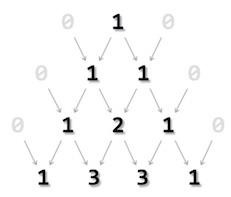# How to print integers in the form of Pascal triangle using C?

Pascal's triangle is the representation of integers in the form of a triangle. One of the famous representations of it is with binomial equations. We can use combinations and factorials to achieve this.

## Constructing a Pascal triangle

All values outside the triangle are considered zero (0). The first row is 0 1 0 whereas only 1 acquire a space in Pascal’s triangle, 0s are invisible. The second row is acquired by adding (0+1) and (1+0). The output is sandwiched between two zeroes. The process continues till the required level is achieved.

Programmatically, a Pascal triangle is defined as an array constructed by adding adjacent elements in preceding rows.## Program 1

In this program, we will print integers in the form of Pascal Triangle with Using Array −

Live Demo

#include <stdio.h>
int fact(int);
int main(){
int i,rows,j;
printf("enter no of rows :");
scanf("%d",&rows);
for (i = 0; i < rows; i++){
for (j = 0; j <= (rows- i - 2); j++)
printf(" ");
for (j = 0 ; j <= i; j++)
printf("%d ",fact(i)/(fact(j)*fact(i-j)));
printf("");
}
return 0;
}
int fact(int n){
int a;
int sum = 1;
for (a = 1; a <= n; a++)
sum = sum*a;
return sum;
}

## Output

Enter no of rows :5
1
1 1
1 2 1
1 3 3 1
1 4 6 4 1

## Program 2

Here, we will see printing integers in the form of Pascal Triangle Without Using Array

Live Demo

#include<stdio.h>
int main(){
int num,row,i;
printf("Enter the number of rows: ");
scanf("%d",&num);
for(row=1; row<=num; row++){
int a=1;
for(i=1; i<=row; i++){
printf("%d ",a);
a = a * (row-i)/i;
}
printf("");
}
return 0;
}

## Output

Enter the number of rows: 6
1
1 1
1 2 1
1 3 3 1
1 4 6 4 1
1 5 10 10 5 1